# Example WVPlots

#### 2018-04-03

Some example data science plots in R using ggplot2. See https://github.com/WinVector/WVPlots for code/details.

set.seed(34903490)
x = rnorm(50)
y = 0.5*x^2 + 2*x + rnorm(length(x))
frm = data.frame(x=x,y=y,yC=y>=as.numeric(quantile(y,probs=0.8)))
frm$absY <- abs(frm$y)
frm$posY = frm$y > 0

## Scatterplots

Scatterplot with smoothing line through points. Reports the square of the correlation between x and y (R-squared) and its significance.

WVPlots::ScatterHist(frm, "x", "y", title="Example Fit")
## geom_smooth() using method = 'loess'
## geom_smooth() using method = 'loess'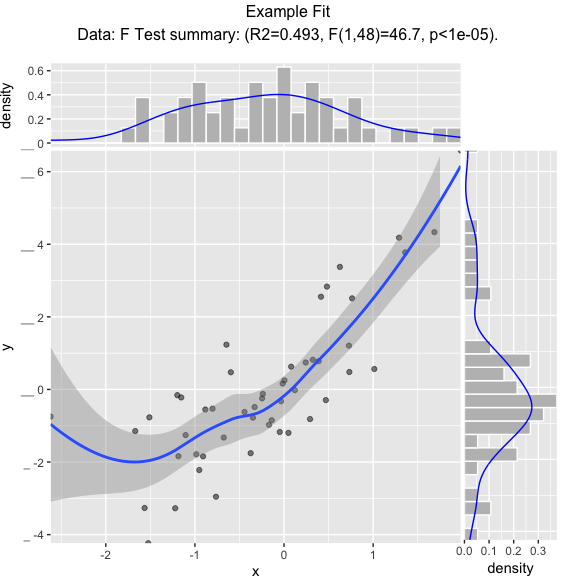Scatterplot with best linear fit through points. Reports the R-squared and significance of the linear fit.

WVPlots::ScatterHist(frm, "x", "y", smoothmethod="lm",
title="Example Linear Fit", annot_size=2)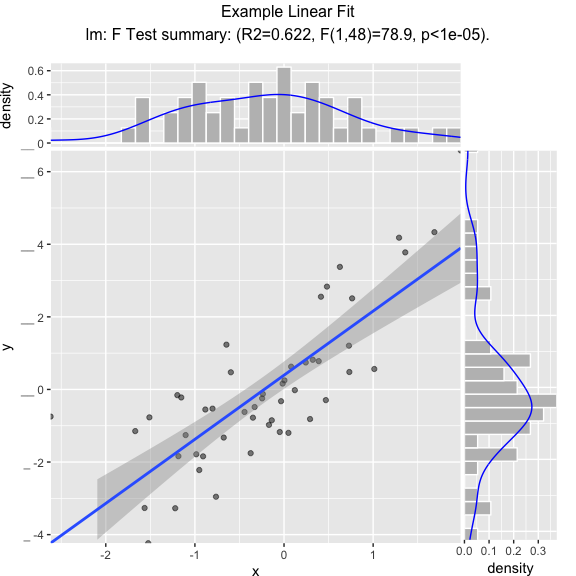Scatterplot compared to the line x = y. Reports the square of the correlation between x and y (R-squared) and its significance.

WVPlots::ScatterHist(frm, "x", "y", smoothmethod="identity",
title="Example Relation Plot", annot_size=2)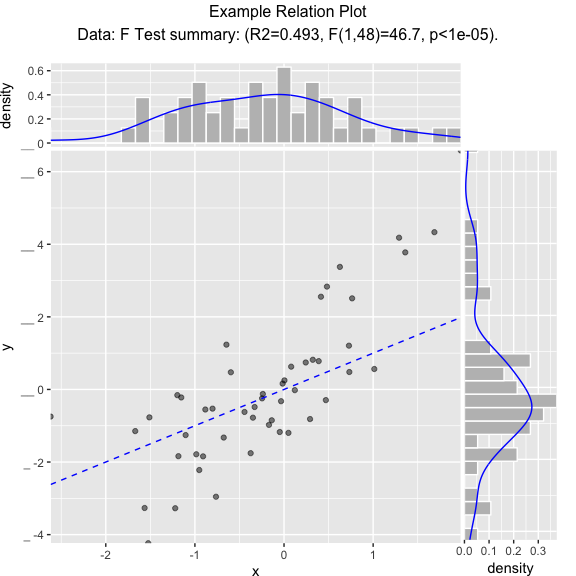Scatterplot of (x, y) color-coded by category/group, with marginal distributions of x and y conditioned on group.

set.seed(34903490)
fmScatterHistC = data.frame(x=rnorm(50),y=rnorm(50))
fmScatterHistC$cat <- fmScatterHistC$x+fmScatterHistC$y>0 WVPlots::ScatterHistC(fmScatterHistC, "x", "y", "cat", title="Example Conditional Distribution")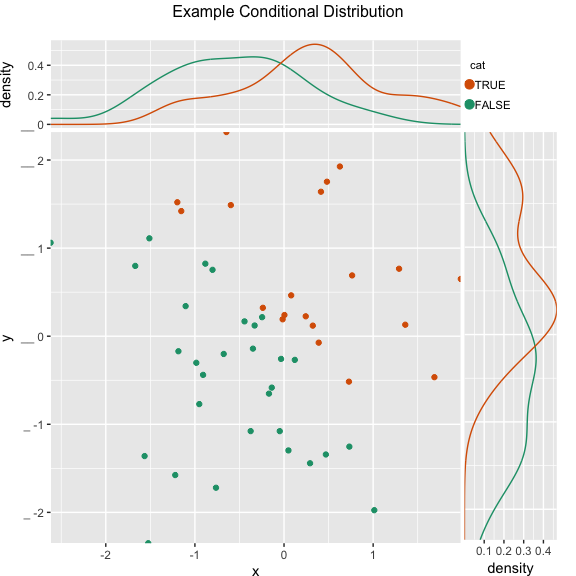Scatterplot of (x, y) color-coded by discretized z. The continuous variable z is binned into three groups, and then plotted as by ScatterHistC set.seed(34903490) frmScatterHistN = data.frame(x=rnorm(50),y=rnorm(50)) frmScatterHistN$z <- frmScatterHistN$x+frmScatterHistN$y
WVPlots::ScatterHistN(frmScatterHistN, "x", "y", "z", title="Example Joint Distribution")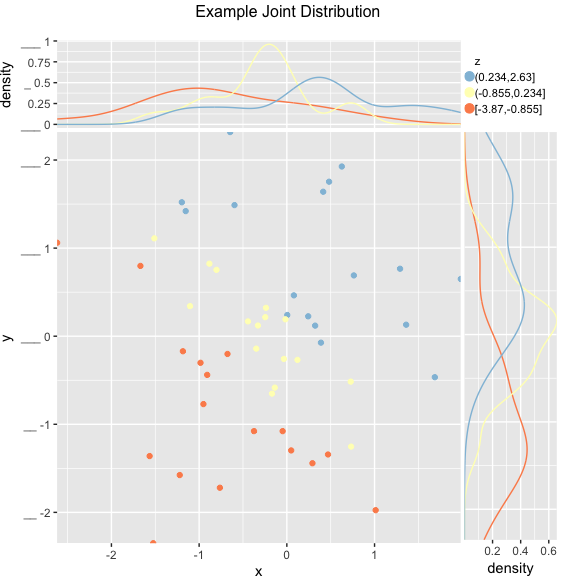Plot the relationship y as a function of x with a smoothing curve that estimates $$E[y | x]$$. If y is a 0/1 variable as below (binary classification, where 1 is the target class), then the smoothing curve estimates $$P(y | x)$$. Since $$y \in \{0,1\}$$ with $$y$$ intended to be monotone in $$x$$ is the most common use of this graph, BinaryYScatterPlot uses a glm smoother by default (use_glm=TRUE, this is essentially Platt scaling), as the best estimate of $$P(y | x)$$.

WVPlots::BinaryYScatterPlot(frm, "x", "posY", use_glm=FALSE,
title="Example 'Probability of Y' Plot (ggplot2 smoothing)")
## geom_smooth() using method = 'loess'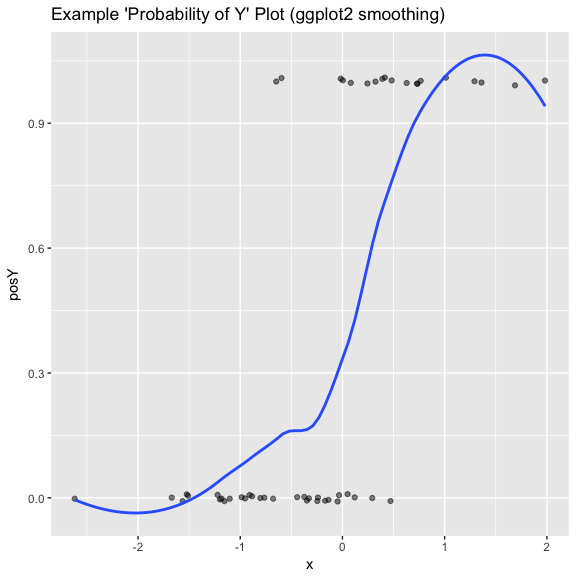WVPlots::BinaryYScatterPlot(frm, "x", "posY", use_glm=TRUE,
title="Example 'Probability of Y' Plot (GLM smoothing)")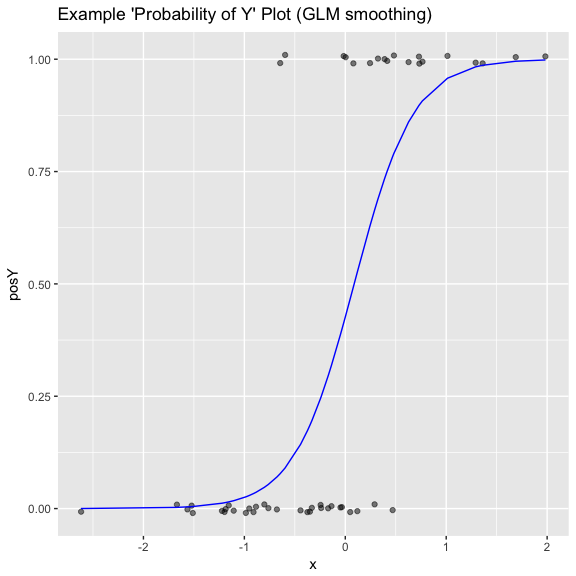## Gain Curves

set.seed(34903490)
y = abs(rnorm(20)) + 0.1
x = abs(y + 0.5*rnorm(20))

frm = data.frame(model=x, value=y)

frm$costs=1 frm$costs=5
frm$rate = with(frm, value/costs) frm$isValuable = (frm$value >= as.numeric(quantile(frm$value, probs=0.8)))

Basic curve: each item “costs” the same. The wizard sorts by true value, the x axis sorts by the model, and plots the fraction of the total population.

WVPlots::GainCurvePlot(frm, "model", "value", title="Example Continuous Gain Curve")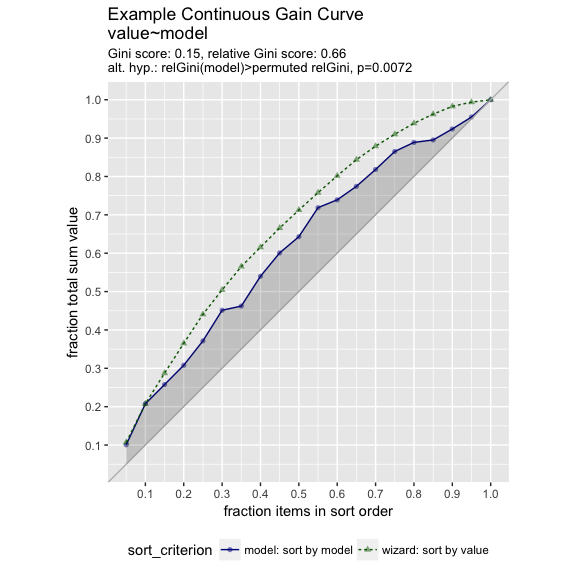We can annotate a point of the model at a specific x value

gainx = 0.10  # get the top 10% most valuable points as sorted by the model

# make a function to calculate the label for the annotated point
labelfun = function(gx, gy) {
pctx = gx*100
pcty = gy*100

paste("The top ", pctx, "% most valuable points by the model\n",
"are ", pcty, "% of total actual value", sep='')
}

WVPlots::GainCurvePlotWithNotation(frm, "model", "value",
title="Example Gain Curve with annotation",
gainx=gainx,labelfun=labelfun)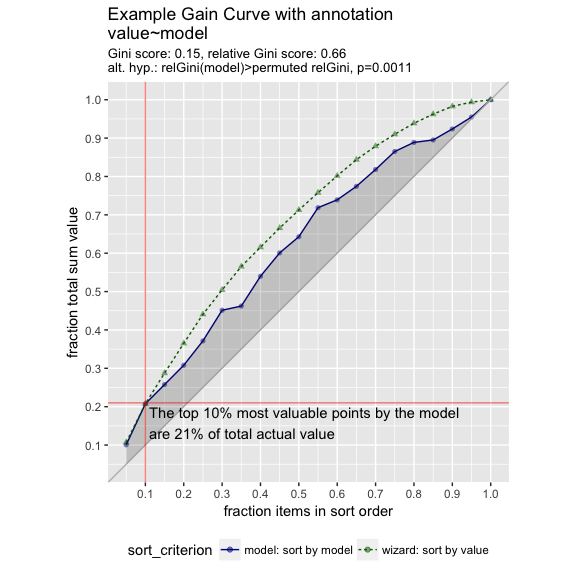When the x values have different costs, take that into account in the gain curve. The wizard now sorts by value/cost, and the x axis is sorted by the model, but plots the fraction of total cost, rather than total count.

WVPlots::GainCurvePlotC(frm, "model", "costs", "value", title="Example Continuous Gain CurveC")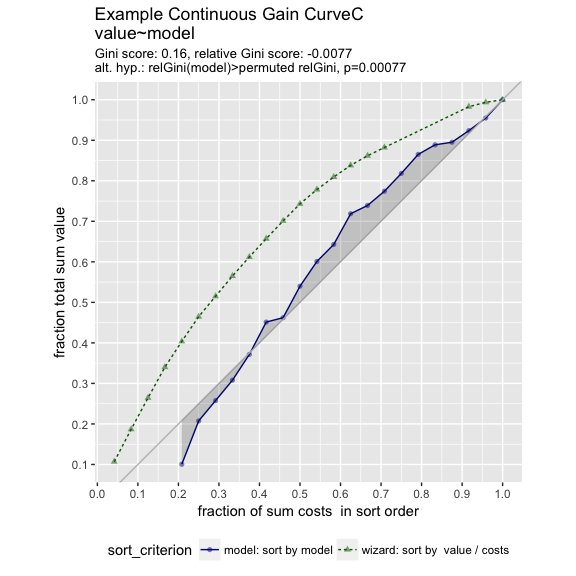## ROC Plots

WVPlots::ROCPlot(frm, "model", "isValuable", TRUE, title="Example ROC plot")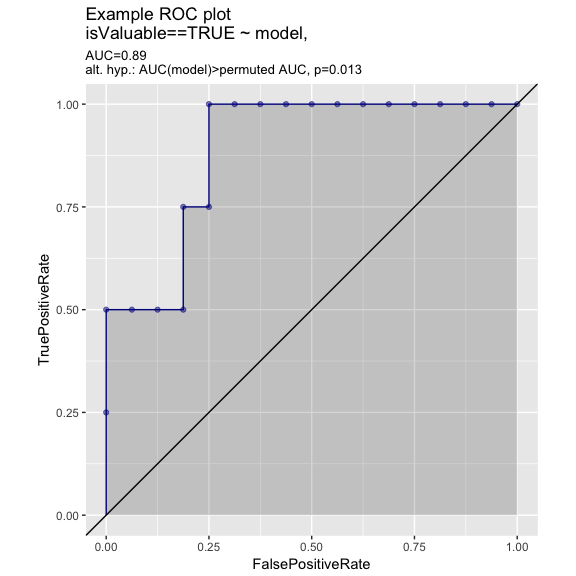set.seed(34903490)
x1 = rnorm(50)
x2 = rnorm(length(x1))
y = 0.2*x2^2 + 0.5*x2 + x1 + rnorm(length(x1))
frmP = data.frame(x1=x1,x2=x2,yC=y>=as.numeric(quantile(y,probs=0.8)))
# WVPlots::ROCPlot(frmP, "x1", "yC", TRUE, title="Example ROC plot")
# WVPlots::ROCPlot(frmP, "x2", "yC", TRUE, title="Example ROC plot")
WVPlots::ROCPlotPair(frmP, "x1", "x2", "yC", TRUE, title="Example ROC pair plot")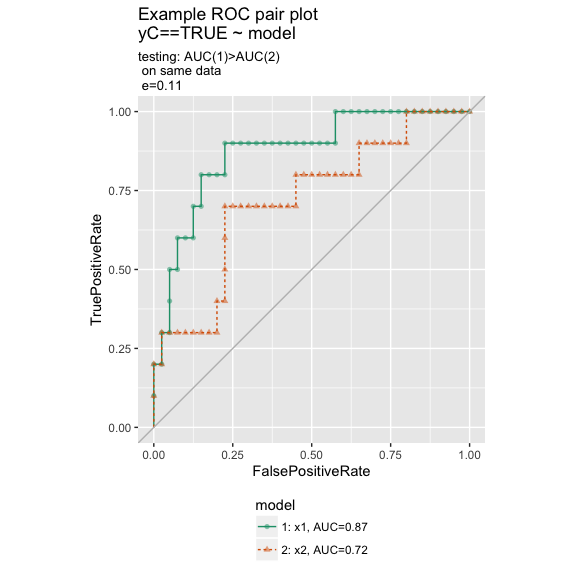## Precision-Recall Plot

WVPlots::PRPlot(frm, "model", "isValuable", TRUE, title="Example Precision-Recall plot")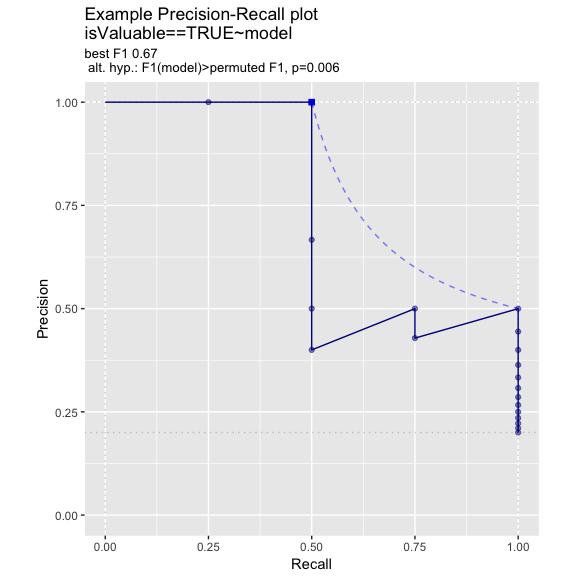## Double Density Plot

WVPlots::DoubleDensityPlot(frm, "model", "isValuable", title="Example double density plot")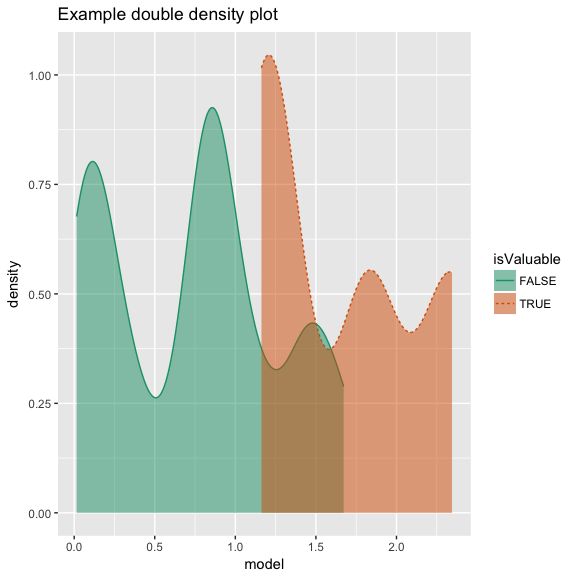## Double Histogram Plot

WVPlots::DoubleHistogramPlot(frm, "model", "isValuable", title="Example double histogram plot")